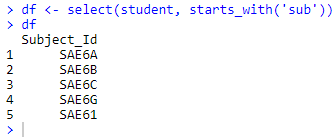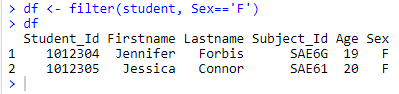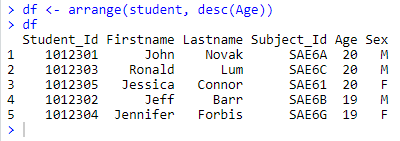Search

# Data Manipulation using DPLYR : Part 1

In this blog, you will learn how to easily perform data manipulation using R software. We’ll use mainly the popular dplyr R package, which contains important R functions to carry out easily your data manipulation. The dplyr package(written by Hadley Wickham) provides us with several functions that facilitate the manipulation of data frames in R. Some of the most useful include:

1. The select Function: facilitates the selection of records (rows)

2. The filter Function: facilitates the selection of variables (columns)

3. The arrange Function: facilitates the ordering of records

4. The mutate Function: facilitates the creation of new variables

5. The rename Function: facilitates the renaming of variables

6. The summarize Function: facilitate the summarization of variables

At the end of this blog, you will be familiar with data manipulation tools and approaches that will allow you to efficiently manipulate data.

# What is Data Manipulation ?

If you are still confused with this ‘term’, let me explain it to you. Data Manipulation is a loosely used term with ‘Data Exploration’. It involves ‘manipulating’ data using available set of variables. This is done to enhance accuracy and precision associated with data.

Actually, the data collection process can have many loopholes. There are various uncontrollable factors which lead to inaccuracy in data such as mental situation of respondents, personal biases, difference / error in readings of machines etc. To mitigate these inaccuracies, data manipulation is done to increase the possible (highest) accuracy in data. At times, this stage is also known as data wrangling or data cleaning.

# Required R package

First, you need to install the dplyr package and load the dplyr library then after you can able to perform the following data manipulation functions.

```install.packages('dplyr')
library(dplyr)```

# Demo Datasets

```student <- data.frame(Student_Id = c(1012301, 1012302, 1012303,          1012304, 1012305),
Firstname = c('John', 'Jeff', 'Ronald', 'Jennifer', 'Jessica'),
Lastname = c('Novak', 'Barr', 'Lum', 'Forbis', 'Connor'),
Subject_Id = c('SAE6A', 'SAE6B', 'SAE6C', 'SAE6G', 'SAE61'),
Age = c(20, 19, 20, 19, 20),
Sex = c('M', 'M', 'M', 'F', 'F'))
print(student)```

Output:# 1. The select Function

The select function allows us to choose the columns to keep within a dataset. This can be done by simply specifying the column names (or numbers) to retain. You can perform data manipulations on either dataframe or CSV file.

Now, you can choose any number of columns using select function. Here, columns 1 to 3 and 5 columns are chosen using both column name and number which shows in below snippet:You can use negatives to select columns to drop:There are a number of additional supporting functions you can use in order to identify columns to select or omit, such as “contains”, “starts_with” and “ends_with” :# 2. The filter Function

The filter function allows us to choose specific rows from a data frame. You achieve this by specifying a logical statement:# 3. The arrange Function

The arrange function allows us to sort the data on 1 or more variables. You provide values which specify the variables by which to sort in ascending order:You can use the desc function to specify that a variable be sorted in descending order:# 4. The mutate Function

You can create new variables within a data frame using the mutate function:Tip: the ifelse function can be used for conditional logic when creating variables.# 5. The rename Function

The rename function provides a neat and highly readable way to rename columns:You can also rename multiple variables at once:

Tip: The new name is on the left and the old name is on the right.# 6. The summarize Function

Often when analyzing a dataset we want to calculate summary statistics; You can do this with the summarize function, in conjunction with several basic summary functions:

• Standard summaries such as mean, median, min, max etc.

• Additional functions provided by dplyr: n, n_distinct

• Sums of logicals, such as sum(x > 10)If you have missing data we can add an option na.rm = TRUE that will find the summary value even if there are missing values.Notice that the default column names are equal to the call that was made. You can replace these by specifying a new column name:# Summary

In this blog on data manipulation in R, we discussed the functions of manipulation of data in R. The dplyr package provides us with several functions that facilitate the manipulation of data (e.g., select, filter, arrange, mutate, summarize, rename).

## When calling the functions:

• The first argument is the input data frame,

• The remaining arguments describe what to do to the data frame

• The function outputs a data frame.